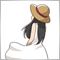# Kama Indicator59

Good morning, I am attaching my KAMA indicator to everyone, kindly tell me where am I wrong ?, the indicator should turn red if the price is below the Kama, and green if the price is above the Kama. Thank you all
Files:603

```//+------------------------------------------------------------------+
//|                                               Kama_Antonello.mq4 |
//|                                                        Antonello |
//|                                             https://www.mql5.com |
//+------------------------------------------------------------------+
#property version   "1.00"
#property strict
#property indicator_chart_window
#property indicator_buffers 2
#property indicator_plots   2
//--- plot KamaSell
#property indicator_label1  "KamaSell"
#property indicator_type1   DRAW_LINE
#property indicator_color1  clrRed
#property indicator_style1  STYLE_SOLID
#property indicator_width1  1
#property indicator_type2   DRAW_LINE
#property indicator_color2  clrGreen
#property indicator_style2  STYLE_SOLID
#property indicator_width2  1
//--- input parameters
input int      PeriodoKama     = 10;
input double   PeriodoEMA_Fast = 2;
input double   PeriodoEMA_Slow = 30;
//--- indicator buffers
double         KamaSellBuffer[];
double         fastest_SC;
double         slowest_SC;

/*
double         ER = 0;
double         Change = 0; //ABS(CLOSE-CLOSE(PERIODOKAMA))
double         Volatility = 0; //SumPeriodoKama(ABS(CLOSE-PRIOR CLOSE))
double         SC = 0;         //[ER*(fastest SC-slowest SC)+slowest SC]2
double         Kama = 0;        //Prior Kama+SC*(PRICE-PRIOR KAMA)
double         fastest_SC = 2 / (PeriodoEMA_Fast + 1);
double         slowest_SC = 2 / (PeriodoEMA_Slow + 1);
*/

//+------------------------------------------------------------------+
//| Custom indicator initialization function                         |
//+------------------------------------------------------------------+
int OnInit()
{
//--- indicator buffers mapping
SetIndexBuffer(0,KamaSellBuffer);

fastest_SC = 2.0 / (PeriodoEMA_Fast + 1.0);
slowest_SC = 2.0 / (PeriodoEMA_Slow + 1.0);
//---
return(INIT_SUCCEEDED);
}
//+------------------------------------------------------------------+
//| Custom indicator iteration function                              |
//+------------------------------------------------------------------+
int OnCalculate(const int rates_total,
const int prev_calculated,
const datetime &time[],
const double &open[],
const double &high[],
const double &low[],
const double &close[],
const long &tick_volume[],
const long &volume[],
{
//---
//int counted_bars = IndicatorCounted();
//if (counted_bars > 0) counted_bars--;
//int limit = Bars - counted_bars;

int i,limit;

if(prev_calculated==0)
limit=rates_total-(PeriodoKama+1);
else
limit=rates_total-prev_calculated;

//for (int i=limit-1; i>0; i--)
//for (int i = 30 - 1; i > 0; i--)
for(i=limit; i>=0; i--)
{
//Change = MathAbs(iClose(Symbol(), 0, i) - iClose(Symbol(), 0, i + PeriodoKama));
double Change=MathAbs(close[i]-close[i+PeriodoKama]);
double Volatility=0;
double ER=1;

for(int x=0; x<PeriodoKama; x++)
{
//Volatility += MathAbs(iClose(Symbol(), 0, i + x) - iClose(Symbol(), 0, i + x + 1));
Volatility+=MathAbs(close[i+x]-close[i+x+1]);
}

if(Volatility>0)
ER=Change/Volatility;

//SC = MathPow(ER * (fastest_SC - slowest_SC) + slowest_SC, 2);
double SC=MathPow((ER *(fastest_SC-slowest_SC)+slowest_SC),2);
//double Kama = iClose(Symbol(), 0, i) + SC * (iClose(Symbol(), 0, i) - Kama);
KamaSellBuffer[i]=KamaSellBuffer[i+1]+SC *(close[i]-KamaSellBuffer[i+1]);

//if(Close[i]>Kama)
if(close[i]>KamaSellBuffer[i])
{
}
else
{
//KamaSellBuffer[i] = Kama;
}
}
//--- return value of prev_calculated for next call
return(rates_total);
}
//+------------------------------------------------------------------+
```59

Thank you very much now I try it.21958

Antonello74: the indicator should turn red
1. Why did you post your MT4 question in the Root / MT5 Indicators section instead of the MQL4 section, (bottom of the Root page?)
General rules and best pratices of the Forum. - General - MQL5 programming forum
Next time post in the correct place. The moderators will likely move this thread there soon.

2. If you assign to one color buffer, make the other color buffer empty.

3. Connect the lines on a color change. Buffer[i]=value[i]; if(Buffer[i+1]==EMPTY_VALUE) Buffer[i+1]=value[i+1].
HOW CAN I hide CONNECTION lines of plots? (ttt) - MQL4 and MetaTrader 4 - MQL4 programming forum

4. I use three buffers. One contains the values, has the proper label for the Data Window and pop up, but uses color set to CLR_NONE, clrNONE so not shown on chart. The other two have proper colors, but with SetIndexLabel(i, NULL) so they don't show in Data Window.59

whroeder1:
1. Why did you post your MT4 question in the Root / MT5 Indicators section instead of the MQL4 section, (bottom of the Root page?)
General rules and best pratices of the Forum. - General - MQL5 programming forum
Next time post in the correct place. The moderators will likely move this thread there soon.

2. If you assign to one color buffer, make the other color buffer empty.

3. Connect the lines on a color change. Buffer[i]=value[i]; if(Buffer[i+1]==EMPTY_VALUE) Buffer[i+1]=value[i+1].
HOW CAN I hide CONNECTION lines of plots? (ttt) - MQL4 and MetaTrader 4 - MQL4 programming forum

4. I use three buffers. One contains the values, has the proper label for the Data Window and pop up, but uses color set to CLR_NONE, clrNONE so not shown on chart. The other two have proper colors, but with SetIndexLabel(i, NULL) so they don't show in Data Window.

thank you and sorry for the error.